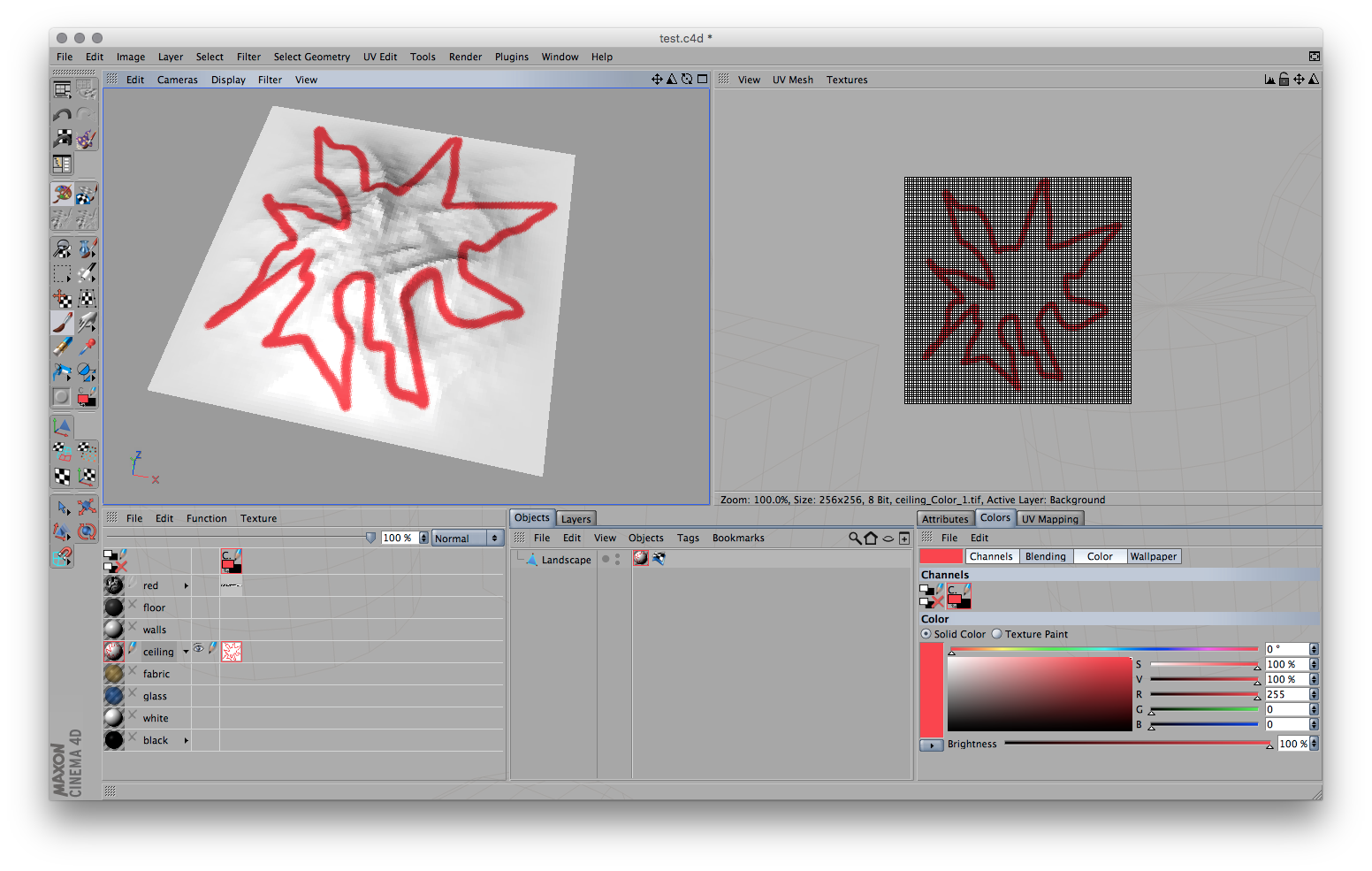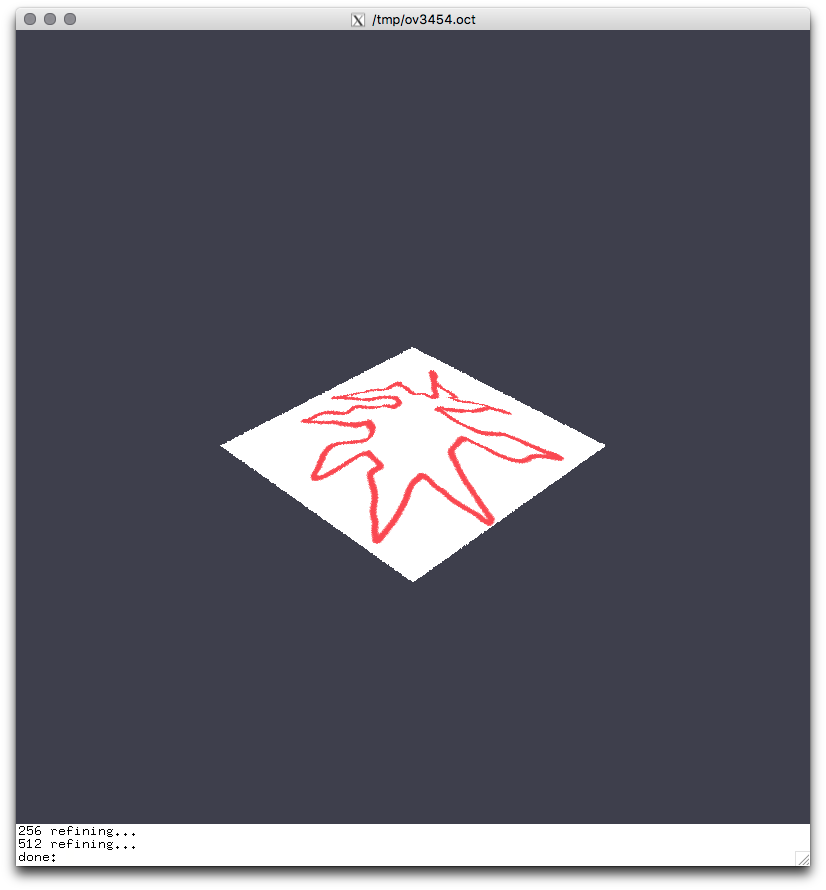Personal tools

You are here: Home / UV Mapping in Radiance

# UV Mapping in RadianceThere is an easy way to use UV mapping, even for procedural texture...just create a UV mapped .obj model and save it.

Then edit the radiance material file and use:

```obj2mesh -a model.mat model.obj > model.mesh
```

Now load the mesh with the primitive mesh in a radiance file and save as model.rad

```void mesh id
1 model.mesh
0
0
```

Notes:

1. Light can be placed in a mesh but it will not not work.
2. Meshes are frozen. Any change to the OBJ model will require regeneration of the mesh.

To attach a pattern (tif image) you need first to convert it to Radiance HDR:

```ra_tiff -r image.tif image.hdr
```

This generally works but may have problems with more exotic tiffs; just use a plain type.

Now you need to get the ratio between horizontal and vertical image size:

```getinfo -d image.hdr  | rcalc -e '\$1=\$5/\$3'
```

Note that this number, (W/H), must to be > 1, so if the ratio is 0.8 you need to consider 1/0.8 (i.e. H/W) instead; This image ratio is referred to as R in the following notes.

### Let's define a few things

The image scale is:
Su,v
1 = 100%, 0.5 = 50% and so on
If you need to translate, in fraction of u,v the parameter is:
Tu,v
1 = 100%, 0.5 = 50% and so on
The image ratio is defined by two values, one is a unit (1) and the other the previous R value:
Ru, Rv
thus 1,R or R,1

The material definition, verbose style, will be:

```void colorpict image
7 red green blue image.hdr . R*((Lu-Tu)/Su-floor((Lu-Tu)/Su)) 1*((Lv-Tv)/Sv-floor((Lv-Tv)/Sv))
0
0
```

or (note that Ru and Rv are swapped):

```void colorpict image
7 red green blue image.hdr . 1*((Lu-Tu)/Su-floor((Lu-Tu)/Su)) R*((Lv-Tv)/Sv-floor((Lv-Tv)/Sv))
0
0
```

A material definition, with indexes is as follows:

```void colorpict image
7 red green blue image.hdr  .  A1*((Lu-A3)/A5-floor((Lu-A3)/A5))  A2*((Lv-A4)/A6-floor((Lv-A4)/A6))
0
6 Ru Rv Tu Tv Su Sv
# edit the Ry, Rv Tu Tv etc. with the values desired.
# 6 A1 A2 A3 A4 A5 A6
```

This is a color layer which needs to be used in conjunction with a base material such as:

```image plastic ceiling
0
0
5 1 1 1 0 0
```

### New possibilities

Once you have Lu and Lv defined you can use them instead of Px Py Pz and all procedural patterns, wood, marble, waves are available for any surface in Radiance.

by – last modified Jun 18, 2016 04:21 PM